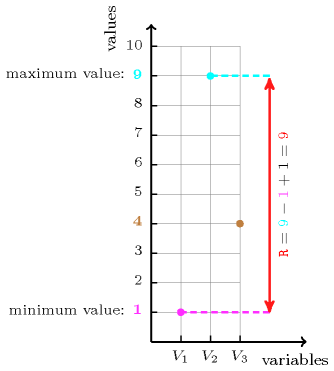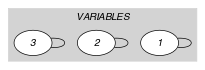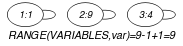## 5.330. range_ctr

Origin

Arithmetic constraint.

Constraint

$\mathrm{𝚛𝚊𝚗𝚐𝚎}_\mathrm{𝚌𝚝𝚛}\left(\mathrm{𝚅𝙰𝚁𝙸𝙰𝙱𝙻𝙴𝚂},\mathrm{𝙲𝚃𝚁},𝚁\right)$

Arguments
 $\mathrm{𝚅𝙰𝚁𝙸𝙰𝙱𝙻𝙴𝚂}$ $\mathrm{𝚌𝚘𝚕𝚕𝚎𝚌𝚝𝚒𝚘𝚗}\left(\mathrm{𝚟𝚊𝚛}-\mathrm{𝚍𝚟𝚊𝚛}\right)$ $\mathrm{𝙲𝚃𝚁}$ $\mathrm{𝚊𝚝𝚘𝚖}$ $𝚁$ $\mathrm{𝚍𝚟𝚊𝚛}$
Restrictions
 $|\mathrm{𝚅𝙰𝚁𝙸𝙰𝙱𝙻𝙴𝚂}|>0$ $\mathrm{𝚛𝚎𝚚𝚞𝚒𝚛𝚎𝚍}$$\left(\mathrm{𝚅𝙰𝚁𝙸𝙰𝙱𝙻𝙴𝚂},\mathrm{𝚟𝚊𝚛}\right)$ $\mathrm{𝙲𝚃𝚁}\in \left[=,\ne ,<,\ge ,>,\le \right]$
Purpose

Constraint the difference between the maximum value and the minimum value of a set of domain variables. More precisely, let $\mathrm{𝚁𝙰𝙽𝙶𝙴}$ denote the difference between the largest and the smallest variables of the $\mathrm{𝚅𝙰𝚁𝙸𝙰𝙱𝙻𝙴𝚂}$ collection plus one. Enforce the following constraint to hold: $\mathrm{𝚁𝙰𝙽𝙶𝙴}\mathrm{𝙲𝚃𝚁}𝚁$.

Example
$\left(〈1,9,4〉,=,9\right)$

The $\mathrm{𝚛𝚊𝚗𝚐𝚎}_\mathrm{𝚌𝚝𝚛}$ constraint holds since $max\left(1,9,4\right)-min\left(1,9,4\right)+1$ is equal (i.e., $\mathrm{𝙲𝚃𝚁}$ is set to $=$) to its last argument $𝚁=9$.

##### Figure 5.330.1. Illustration of the Example slot: three variables respectively fixed to values 1, 9 and 4, and their corresponding range $𝚁=9$Typical
 $|\mathrm{𝚅𝙰𝚁𝙸𝙰𝙱𝙻𝙴𝚂}|>1$ $\mathrm{𝚛𝚊𝚗𝚐𝚎}$$\left(\mathrm{𝚅𝙰𝚁𝙸𝙰𝙱𝙻𝙴𝚂}.\mathrm{𝚟𝚊𝚛}\right)>1$ $\mathrm{𝙲𝚃𝚁}\in \left[=,<,\ge ,>,\le \right]$
Symmetries
• Items of $\mathrm{𝚅𝙰𝚁𝙸𝙰𝙱𝙻𝙴𝚂}$ are permutable.

• All occurrences of two distinct values of $\mathrm{𝚅𝙰𝚁𝙸𝙰𝙱𝙻𝙴𝚂}.\mathrm{𝚟𝚊𝚛}$ can be swapped.

• One and the same constant can be added to the $\mathrm{𝚟𝚊𝚛}$ attribute of all items of $\mathrm{𝚅𝙰𝚁𝙸𝙰𝙱𝙻𝙴𝚂}$.

Arg. properties
• Contractible wrt. $\mathrm{𝚅𝙰𝚁𝙸𝙰𝙱𝙻𝙴𝚂}$ when $\mathrm{𝙲𝚃𝚁}\in \left[<,\le \right]$.

• Extensible wrt. $\mathrm{𝚅𝙰𝚁𝙸𝙰𝙱𝙻𝙴𝚂}$ when $\mathrm{𝙲𝚃𝚁}\in \left[\ge ,>\right]$.

Used in
Keywords
Arc input(s)

$\mathrm{𝚅𝙰𝚁𝙸𝙰𝙱𝙻𝙴𝚂}$

Arc generator
$\mathrm{𝑆𝐸𝐿𝐹}$$↦\mathrm{𝚌𝚘𝚕𝚕𝚎𝚌𝚝𝚒𝚘𝚗}\left(\mathrm{𝚟𝚊𝚛𝚒𝚊𝚋𝚕𝚎𝚜}\right)$

Arc arity
Arc constraint(s)
$\mathrm{𝚃𝚁𝚄𝙴}$
Graph property(ies)
$\mathrm{𝐑𝐀𝐍𝐆𝐄}$$\left(\mathrm{𝚅𝙰𝚁𝙸𝙰𝙱𝙻𝙴𝚂},\mathrm{𝚟𝚊𝚛}\right)\mathrm{𝙲𝚃𝚁}𝚁$

Graph model

Since we want to keep all the vertices of the initial graph we use the $\mathrm{𝑆𝐸𝐿𝐹}$ arc generator together with the $\mathrm{𝚃𝚁𝚄𝙴}$ arc constraint. This predefined arc constraint always holds.

Parts (A) and (B) of Figure 5.330.2 respectively show the initial and final graph associated with the Example slot. Since we use the $\mathrm{𝚃𝚁𝚄𝙴}$ arc constraint both graphs are identical.

##### Figure 5.330.2. Initial and final graph of the $\mathrm{𝚛𝚊𝚗𝚐𝚎}_\mathrm{𝚌𝚝𝚛}$ constraint(a) (b)